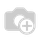# Is it possible to call a function field method from onchange function.

i created a function field tax_amount in sale_order_line. Now i want to call my function field from existing onchange function of product uom in sale order line. How to call a function field function from onchange function and give me example

(or)

Simply i want to compute a sale order amounts(amount_untaxed, amount_tax, amount_total) for each sale order line entries. Can be a solution or idea to achieve thisHi,

Hope you are working with v8. I don't think, you need to override the onchange function.

If your tax_amount depends only on product uom, you can define the tax_amount as a compute field which depends on product_uom. onchange is default in compute fields. If tax_amount depends on more fields, you can include that also. For eg:

`class sale_order_line(models.Model):    _inherit = 'sale.order.line'        tax_amount = fields.Float(compute='_get_tax_amount', string='Tax Amount')        @api.one    @api.depends('product_uom', 'product_id')    def _get_tax_amount(self):                //Your code here that calculates the tax value        self.tax_amount = tax value`Hi,

You can call the function field method from onchange method.

`class sale_order_line(models.Model):    _inherit = 'sale.order.line'        tax_amount = fields.Float(compute='_get_tax_amount', string='Tax Amount')        @api.one    @api.depends('product_uom', 'product_id')    def _get_tax_amount(self):                //Your code here that calculates the tax value        self.tax_amount = tax value`Simply call your function from within the on_change method.

Take care of the scope and use self.function_name.

An example can be found in the hr_holidays module: onchange_date_from calls _get_number_of_days

Regards.

1 CommentYes! You can call a function(Normal) from onchange, but need to call a function _amount_tax_line (function field defined in column of sale.order.line class). A function field column is called when you press Save button but need to call from onchange_product_id function from sale.order.line...